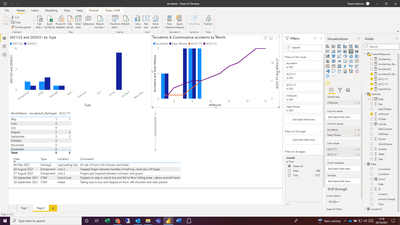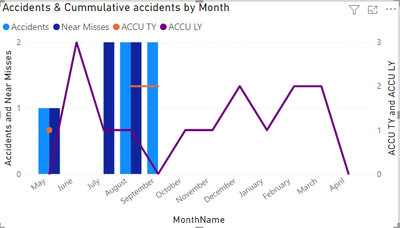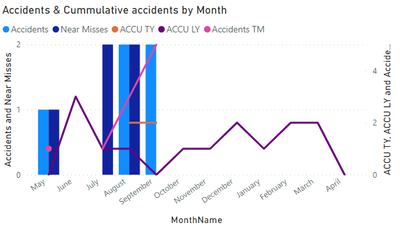cancel
Showing results for
Did you mean:Frequent Visitor

## Running Total

I have a simple data model recording accidents per month and want to create a bar graph showing month accidents and a running total.

Normally this wouldn't be an issue but our Accidents data uses a start month of May i have a custom column to give a value of 1 to May, 2 to June etc. If i create the acumulative total on this value everything works fine:However i'd rather show the month names rather than number. If i chage the axis the date changes and only shows the actual figure:The month Name isn't a numerical fielf for me to use in the accumulation calulation. Is there a way to do this?My measure is:

ACCU TY =
VAR MaxW = calculate(max('Calendar'[HSMonth]),'Calendar'[HSYear] = 2021)
return CALCULATE(
CustomMeasures[AccidentsTy],
FILTER(
ALLSELECTED('Calendar'[HSMonth]),
ISONORAFTER('Calendar'[HSMonth], MaxW, DESC)
)
)
4 REPLIES 4Super User

Try this:

``````Meausre=
CALCULATE(
[AccidentsTy],
FILTER(
all(Calendar),Calendar(date)<=max(calendar(date)
)
)``````

If this post helps, please consider accepting it as the solution to help the other members find it more quickly.Frequent Visitor

Thanks Vahid, that just gives me a complete total which is the same each month:It shows the total rather than a running total on both graphs (MonthName and HSMonth)Super User

It would be better if you shared a sample of your data or the PBIX file.

BTE, try this:

``````Meausre=
CALCULATE(
[AccidentsTy],
FILTER(
all(Data),Data(date)<=max(calendar(date)
)
)``````

If this post helps, please consider accepting it as the solution to help the other members find it more quickly.Frequent Visitor

Thanks Vahid,

Almost there. is there a way to account for zero values. Currently it looks like this:If i use the measure that adds 0 values for the barchart i get the result below: